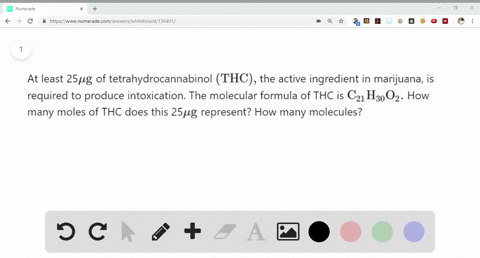Sign up for our free STEM online summer camps starting June 1st!View Summer Courses### At least 25$\mu \mathrm{g}$ of tetrahydrocannabin…

05:38University of New Mexico

Need more help? Fill out this quick form to get professional live tutoring.

Get live tutoring
Problem 43

The allowable concentration level of vinyl chloride, $\mathrm{C}_{2} \mathrm{H}_{3} \mathrm{Cl}$ , in the atmosphere in a chemical plant is $2.0 \times 10^{-6} \mathrm{g} / \mathrm{L}$ . How many moles of vinyl chloride in each liter does this represent? How many molecules per liter?

3.2$\cdot 10^{-8} \mathrm{mol} / \mathrm{L}$
1.93$\cdot 10^{16} \mathrm{molecules} / \mathrm{L}$

## Discussion

You must be signed in to discuss.

## Video Transcript

first off, all we need to calculate the Mullah Omar's off. See, too. It's three see here, which can be created. US. Two multiplied with 12 for carbon less, three multiplied with one for hydrogen plus one multiplied with 35 point four for chlorine. Giving us the Mullah Omar's as 62 point four. Yeah, 62.4 grams. Our move for the world. We need to calculate the number off molds for leader off atmosphere. 62.4 grams will be equal to one mole, hence 2.0 into 10 days. Toe minus six. Ground will be equal to three point 20 into 10 days. Tobar minus eight mon. This calculation has been done using the unitary method to calculate the number off molecules leader off atmosphere. We know that one more will have 6.2 into 10 days to buy 23 molecules for though thus 3.20 into 10 days. Tobar minus eight. Moles will have 1.93 into tenders. Tobar, 16 molecules for leader